### Numerical Methods–Unit 2–2 Marks with Answers

Anna University

Numerical Methods

UNIT-II

INTERPOLATION AND APPROXIMATIONT

1. Explain briefly Interpolation.

Ans: Interpolation is the process of computing the values of a function for any value of the independent variable within an interval for which some values are given.

2. Definition of Interpolation and extrapolation.

Ans: Interpolation: It is the process of finding the intermediate values of a function from a set of its values specific points given in a tabulated form. The process of computing y corresponding to x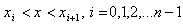is interpolation.

Extrapolation: If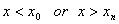then the process is called extrapolation.

3. State Newton’s Forward interpolation formula.4. State Newton’s Backward interpolation formula.5. Error in Newton’s forward: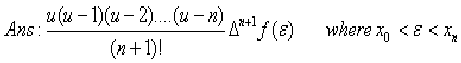6. Error in Newton’s Backward: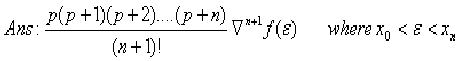7. State Newton’s divided difference formula.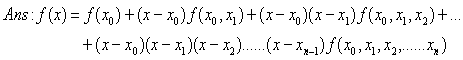8. Show that the divided differences are symmetrical in their arguments.9. Show that divided difference operator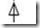is linear.

10. Divided difference table:
 X Y X0 X1 X2 X3 Y0 Y1 Y2 Y311. Write Lagrangian’s polynomial formula.12. What is the assumption we make when Lagrange’s formula is used?

Ans: It can be used whether the values of x, the independent variable are equally spaced or not whether the difference of y become smaller or not.

13. Write Lagrangian inverse interpolation formula.14. Define Cubic Spline

Ans: Let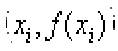, i = 0, 1, 2... n be the given (n +1) pairs of a data. The third order curves employed to connect each pair of data points are called cubic splines. (OR) A smooth polynomial curve is known as cubic spline.

A cubic spline function f(x) w.r.t. the points x0, x1, .....xn is a polynomial of degree three in each interval (xi-1, xi) i = 1, 2, ...n such that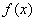,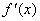and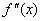are continuous.

15. Write down the formula of Cubic Spline.(OR)